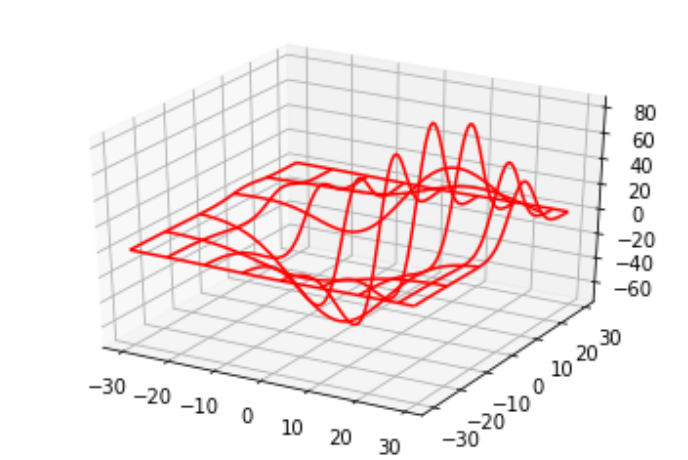# Python – 3D Charts

Python is also capable of creating 3d charts. It involves adding a subplot to an existing two-dimensional plot and assigning the projection parameter as 3d.

## Drawing a 3D Plot

3dPlot is drawn by mpl_toolkits.mplot3d to add a subplot to an existing 2d plot.

```from mpl_toolkits.mplot3d import axes3d
import matplotlib.pyplot as plt

chart = plt.figure()